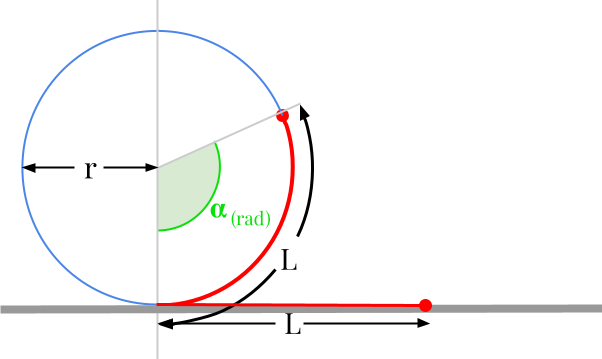Question #815

# What is the formula to convert meters to radians?

Merged questions

You can't actually convert meters to radians. Radian is a measure of angle, while meters is a unit of distance. There is an homogeneity issue between the two quantities.However, there are many mechanical systems that transform linear motion into rotary motion. The most obvious system is the wheel: the rotation of the wheel moves the vehicle forward and vice versa. In this case, the formula governing such a system and allowing to transform a displacement given in meters into a rotation of an angle expressed in radians is given by:

﻿﻿

﻿﻿

with :

• ﻿﻿ the distance or length expressed in metres [m]
• ﻿﻿ is the radius expressed in metres [m]